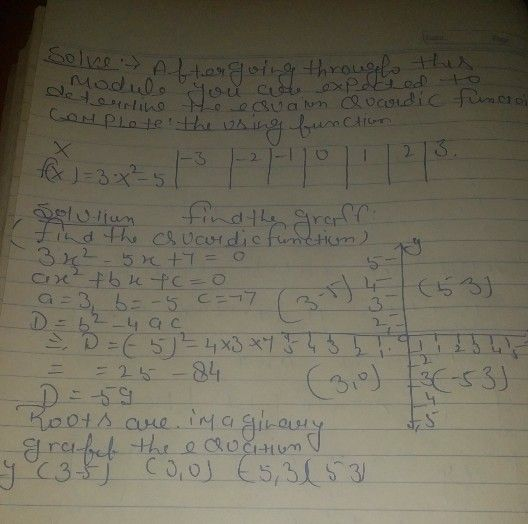Symbol
ProblemLesson $\left(0$ $4n=1$ going through this module, $y0$ are expected to determines the equation of a quadratic function given: $n$ (a) $a$ table of values; $1b$ graph; (c) zeros and solves problems involving quadratic functions. Complete the table using the function of x. $\dfrac {x} {f\left(x\right)=3x^{2}-5}$ $-$ $-3$ $-2$ $-1$ $0$ $1$ $2$ $3$ $-$ $-$
7th-9th grade
Algebra
Search count: 113
SolutionQanda teacher - Devkiran